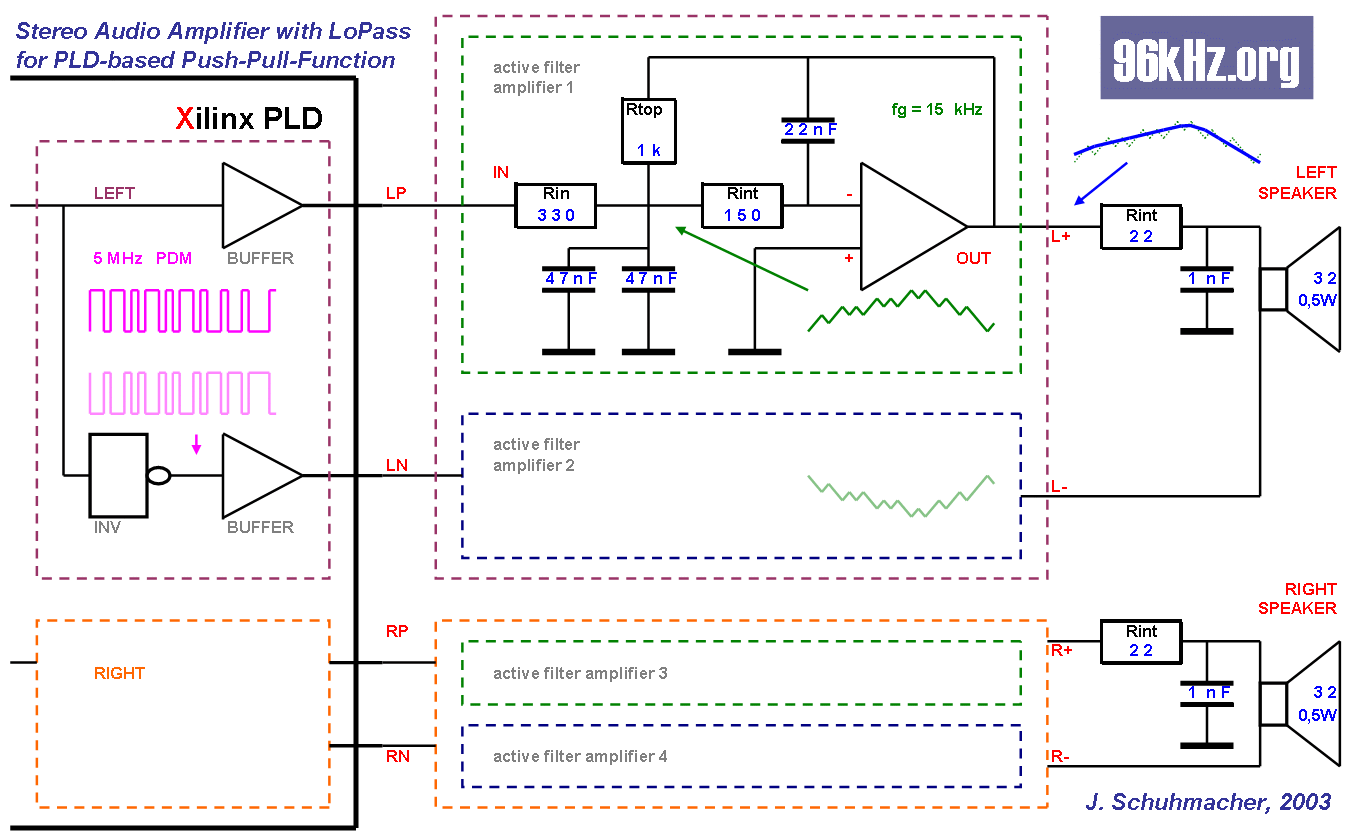96 kHz.org Advanced Audio Recording Pulse Density Modulation The method of PDM is a common technique to generate a one bit serial data stream from a parallel digital vector, to represent it's analog corresponding value at only one pin. This is often required to transport digital data or turn it into an analog value without a DAC. In fact PDM is a kind of DAC as explained here: PDM for Audio  Delta Sigma Conversion  As explained in the article, delta sigma conversion generates an ideal one bit output of the digital value which in theory one has to be filtered appropriately to obtain an analog voltage. In the digital domain using FIR-filters this works fine because ideal mathematical algorithms are used. Practically there are some problems:Learn more about the function of this circuit in the former article mono amplifier.  Related Information © 2004 Jürgen Schuhmacher